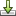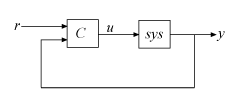# pidTuner

Open PID Tuner for PID tuning

## Syntax

```pidTuner(sys,type) pidTuner(sys,Cbase) pidTuner(sys) pidTuner ```

## Description

`pidTuner(sys,type)` launches the PID Tuner app and designs a controller of type `type` for plant `sys`.

`pidTuner(sys,Cbase)` launches PID Tuner with a baseline controller `Cbase` so that you can compare performance between the designed controller and the baseline controller. If `Cbase` is a `pid`, `pidstd`, `pid2` or `pidstd2` controller object, PID Tuner designs a controller of the same form, type, and discrete integrator formulas as `Cbase`.

`pidTuner(sys)` designs a parallel-form PI controller.

`pidTuner` launches PID Tuner with default plant of 1 and proportional (P) controller of 1.

## Input Arguments

`sys`

Plant model for controller design. `sys` can be:

If the plant has unstable poles, and `sys` is either:

• A `frd` model

• A `ss` model with internal time delays that cannot be converted to I/O delays

then you must specify the number of unstable poles in the plant. To do this, after opening PID Tuner, in the Plant menu, select`Import`. In the Import Linear System dialog box, reimport `sys`, specifying the number of unstable poles where prompted.

`type`

Controller type of the controller to design, specified as a character vector. The term controller type refers to which terms are present in the controller action. For example, a PI controller has only a proportional and an integral term, while a PIDF controller contains proportional, integrator, and filtered derivative terms. `type` can take the values summarized below. For more detailed information about these controller types, see PID Controller Types for Tuning

#### 1-DOF Controllers

• `'P'` — Proportional only

• `'I'` — Integral only

• `'PI'` — Proportional and integral

• `'PD'` — Proportional and derivative

• `'PDF'` — Proportional and derivative with first-order filter on derivative term

• `'PID'` — Proportional, integral, and derivative

• `'PIDF'` — Proportional, integral, and derivative with first-order filter on derivative term

#### 2-DOF Controllers

• `'PI2'` — 2-DOF proportional and integral

• `'PD2'` — 2-DOF proportional and derivative

• `'PDF2'` — 2-DOF proportional and derivative with first-order filter on derivative term

• `'PID2'` — 2-DOF proportional, integral, and derivative

• `'PIDF2'` — 2-DOF proportional, integral, and derivative with first-order filter on derivative term

#### 2-DOF Controllers with Fixed Setpoint Weights

• `'I-PD'` — 2-DOF PID with b = 0, c = 0

• `'I-PDF'` — 2-DOF PIDF with b = 0, c = 0

• `'ID-P'` — 2-DOF PID with b = 0, c = 1

• `'IDF-P'` — 2-DOF PIDF with b = 0, c = 1

• `'PI-D'` — 2-DOF PID with b = 1, c = 0

• `'PI-DF'` — 2-DOF PIDF with b = 1, c = 0

For more detailed information about fixed-setpoint-weight 2-DOF PID controllers, see PID Controller Types for Tuning.

#### Controller Form

When you use the `type` input, PID Tuner designs a controller in parallel form. If you want to design a controller in standard form, Use the input `Cbase` instead of `type`, or select `Standard` from the Form menu. For more information about parallel and standard forms, see the `pid` and `pidstd` reference pages.

If `sys` is a discrete-time model with sample time `Ts`, PID Tuner designs a discrete-time `pid` controller using the `ForwardEuler` discrete integrator formula. To design a controller having a different discrete integrator formula:

• Use the input argument `Cbase` instead of `type`. PID Tuner reads controller type, form, and discrete integrator formulas from the baseline controller `Cbase`.

• In PID Tuner, click Options to open the Controller Options dialog box. Select discrete integrator formulas from the Integral Formula and Derivative Formula menus.

For more information about discrete integrator formulas, see the `pid` and `pidstd` reference pages.

`Cbase`

A dynamic system representing a baseline controller, permitting comparison of the performance of the designed controller to the performance of `Cbase`.

If `Cbase` is a `pid` or `pidstd` object, PID Tuner also uses it to configure the type, form, and discrete integrator formulas of the designed controller. The designed controller:

• Is the type represented by `Cbase`.

• Is a parallel-form controller, if `Cbase` is a `pid` controller object.

• Is a standard-form controller, if `Cbase` is a `pidstd` controller object.

• Is a parallel-form 2-DOF controller, if `Cbase` is a `pid2` controller object.

• Is a standard-form 2-DOF controller, if `Cbase` is a `pidstd2` controller object.

• Has the same `Iformula` and `Dformula` values as `Cbase`. For more information about `Iformula` and `Dformula`, see the `pid` and `pidstd` reference pages .

If `Cbase` is any other dynamic system, PID Tuner designs a parallel-form PI controller. You can change the controller form and type using the Form and Type menus after launching PID Tuner.

## Examples

Interactive PID Tuning of Parallel-Form Controller

Launch PID Tuner to design a parallel-form PIDF controller for a discrete-time plant:

```Gc = zpk([],[-1 -1 -1],1); Gd = c2d(Gc,0.1); % Create discrete-time plant pidTuner(Gd,'pidf') % Launch PID Tuner ```

Interactive PID Tuning of Standard-Form Controller Using Integrator Discretization Method

Design a standard-form PIDF controller using `BackwardEuler` discrete integrator formula:

```Gc = zpk([],[-1 -1 -1],1); Gd = c2d(Gc,0.1); % Create discrete-time plant % Create baseline controller. Cbase = pidstd(1,2,3,4,'Ts',0.1,... 'IFormula','BackwardEuler','DFormula','BackwardEuler') pidTuner(Gd,Cbase) % Launch PID Tuner```

PID Tuner designs a controller for `Gd` having the same form, type, and discrete integrator formulas as `Cbase`. For comparison, you can display the response plots of `Cbase` with the response plots of the designed controller by clicking the Show baseline checkbox in PID Tuner.

## Tips

• If `type` or `Cbase` specifies a one-degree-of-freedom (1-DOF) PID controller, then `pidTuner` designs a controller for the unit feedback loop as illustrated:• If `type` or `Cbase` specifies a two-degree-of-freedom (2-DOF) PID controller, then `pidTuner` designs a 2-DOF controller as in the feedback loop of this illustration:• PID Tuner has a default target phase margin of 60 degrees and automatically tunes the PID gains to balance performance (response time) and robustness (stability margins). Use the Response time or Bandwidth and Phase Margin sliders to tune the controller's performance to your requirements. Increasing performance typically decreases robustness, and vice versa.

• Select response plots from the Response menu to analyze the controller's performance.

• If you provide `Cbase`, check Show baseline to display the response of the baseline controller.

• For more detailed information about using PID Tuner, see Designing PID Controllers with PID Tuner.

• For interactive PID tuning in the Live Editor, see the Tune PID Controller Live Editor task. This task lets you interactively design a PID controller and automatically generates MATLAB® code for your live script.

## Algorithms

For information about the MathWorks® PID tuning algorithm, see PID Tuning Algorithm.

## Alternatives

You can open PID Tuner from the MATLAB desktop, in the Apps tab. When you do so, use the Plant menu in PID Tuner to specify your plant model.

For PID tuning at the command line, use `pidtune`. The `pidtune` command can design a controller for multiple plants at once.

For interactive PID tuning in the Live Editor, see the Tune PID Controller Live Editor task. This task lets you interactively design a PID controller and automatically generates MATLAB code for your live script.

## References

Åström, K. J. and Hägglund, T. Advanced PID Control, Research Triangle Park, NC: Instrumentation, Systems, and Automation Society, 2006.